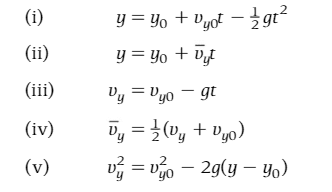# Calculating a ratio in Free Fall motion

• vAhmed

## Homework Statement

A ball is thrown upward with a speed v 1. The ball reaches a maximum height of y=h. What is the ratio of the speed of the ball,v 2 , at y=h/2, to the initial upward speed of the ball,v 1 , at y=0?

## Homework Equations

I attached a picture.We are currently studying the four equations for motions in a straight line.

## The Attempt at a Solution

I know that the acceleration would be constant as a = -g = -9.81m/s^2. And as the ball moves upward the acceleration will slow down the ball due to gravity. The ball was thrown meaning that v1≠ 0. So I set v1 = 9.81 m/s, and v2 = 9.81/2 = 4.9 m/s. Then V2/V1 equals 4.9/9.8 = 0.50. Although I think it doesn't make sense to divide 9.81 by 2. At this point, I couldn't do any better.

#### Attachments

Last edited:

I tried to calculate the velocity at y=0 to divide it by the velocity at y=h/2 but couldn't do it. I also thought of substituting or using imaginary values.
You seen to have removed the template where you were asked to show your work and show applicable equations.

We cannot tell what you have tried unless you tell us.

You seen to have removed the template where you were asked to show your work and show applicable equations.

We cannot tell what you have tried unless you tell us.
Sorry for that, I posted another thread.

I tried to calculate the velocity at y = 0 and y = h/2 but I couldn't. I also thought about substituting imaginary values.
It's not just the template you need. You need to show your work. Tell us what things you have tried. Not just the fact that they've failed.

Last edited:
•CWatters

## Homework Statement

A ball is thrown upward with a speed v 1. The ball reaches a maximum height of y=h. What is the ratio of the speed of the ball,v 2 , at y=h/2, to the initial upward speed of the ball,v 1 , at y=0?

## Homework Equations

I attached a picture.
View attachment 231564
We are currently studying the four equations for motions in a straight line.

## The Attempt at a Solution

I know that the acceleration would be constant as a = -g = -9.81m/s^2. And as the ball moves upward the acceleration will slow down the ball due to gravity. The ball was thrown meaning that v1≠ 0. So I set v1 = 9.81 m/s, and v2 = 9.81/2 = 4.9 m/s. Then V2/V1 equals 4.9/9.8 = 0.50. Although I think it doesn't make sense to divide 9.81 by 2. At this point, I couldn't do any better.
I don't want the answer, I just want to know what should I do to solve it.

It's not just the template you need. You need to show your work. Tell us what things you have tried. Not just the fact that they've failed.